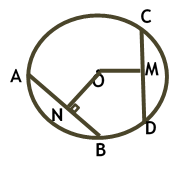Maths / Circles / Equal Chords are Equidistant from the Center of Circle

QUESTION

 In the given circle, if O is the centre of the circle, AB = CD and OM = 4 units, then ON is equal toOPTIONS A. 3 units B. 4 units C. 5 units D. 6 units
Right Option : B

EXPLANATION
Explain TypeExplanation Content
Text

As equal chords are equidistant from the centre and AB = CD and the distancde of CD is 4 units so AB is also at adistance of 4 units

TestimonialsSTUDENT FEEDBACK - Shivam Rana C/o ABHYAS Academy
7th
My experience with Abhyas is very good. I have learnt many things here like vedic maths and reasoning also. Teachers here first take our doubts and then there are assignments to verify our weak points.